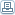# Forex: Pivot Points Calculation Rules

 By: Andrey MoraruThe floor pivot points (the most basic and popular type of pivots) are widely used in Forex trading technical analysis. The main aim of a pivot point is to represent a primary level of support/resistance - the point at which the trend can become bearish or bullish. Levels of resistance and support (from first to third) serve as the additional points of possible trend breakouts or the trend range limits. These are the rules to calculate floor pivot points: Pivot (P) = (H + L + C) / 3 Resistance (R1) = (2 X P) - L R2 = P + H - L R3 = H + 2 X (P - L) Support (S1) = (2 X P) - H S2 = P - H + L S3 = L - 2 X (H - P) Tom DeMark’s pivot points are not as popular as floor pivots, but it is even simpler and can be used to determine the range for a current period trading corridor using the High, Low and Close values of the previous period and the Open value of a current period. To calculate DeMark's pivot points one can use these rules: If Close < Opencurrent Then X = H + 2 X L + C; If Close > Opencurrent Then X = 2 X H + L + C; If Close = Opencurrent Then X = H + L + 2 X C; New High = X / 2 - L; New Low = X / 2 - H Another way to calculate pivot points are Woodie's pivot points. They are very similar to floor pivot points, but are calculated giving more weight to the Close price of the previous time period. The rules to calculate Woodie's pivot points are as follows: Pivot (P) = (H + L + 2 X C) / 4 Resistance (R1) = (2 X P) - L R2 = P + H - L Support (S1) = (2 X P) - H S2 = P - H + L Camarilla pivot points are based on the Camarilla equation method developed by Nick Scott. They are presented as a set of eight levels of support and resistance values without a middle pivot point (which is crucial for floor pivot points). The precise way of calculating these pivot points is somewhat unclear. But more important is that these pivot points can still be calculated and work for all traders. They can be used to set the stop-loss and take-profit orders to automate Forex trading. Use the following rules to calculate Camarilla pivot points: R4 = (H - L) X 1.1 / 2 + C R3 = (H - L) X 1.1 / 4 + C R2 = (H - L) X 1.1 / 6 + C R1 = (H - L) X 1.1 / 12 + C S1 = C - (H - L) X 1.1 / 12 S2 = C - (H - L) X 1.1 / 6 S3 = C - (H - L) X 1.1 / 4 S4 = C - (H - L) X 1.1 / 2
 Foreign Exchange

Most Read Articles On
"Foreign Exchange"SuggestedTop Searches on
 Foreign Exchange

 Share this article :
Click to see more related articles